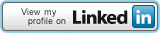Loading...in few seconds...

Click to Call Toll Free: (800) 339-9171
8:00AM EST - 8:00PM EST Mon. to Sat.Search the Repository [Advanced Search]Browse by Category -- Select Category -- Pumps & ThermoSolar PanelsWind TurbineBase Of Systems    - Invereters        - Outback Power        - Magnum Energy    - Microinvertes        - Enpahse Microinverters    - Power Optimizers        - eIQ Energy        - Enphase Microinverter    - Electrical Distribution    - Plant Monitoring        - MNBCM Battery Capacity Meter        - Xantrex Battery Monitor        - PentaMetric Monitoring    - Battery        - GNB Batteries        - Trojan Batteries        - Hawkers Batteries        - Solar One Batteries        - Interstate Batteries        - MK | East Penn | Dekka | Batteries     - Battery Chargers        - IOTA DLS Chargers        - Samlex SEC Series Chargers     - Battery Enclosures        - MidNite Battery Enclosures        - Radiant Solar Technology    - Battery Desulphator    - PV Cables Connectors    - PV Safety Labels    - Wire & CablesSite Assessment    - Daystar Solar Power Meter    - HukseFlux    - Solar Pathfinder    - Watch Dog Weather StationDesign Integration Incentives Product Data    - Warranty    - Flir Thermal Cameras    - Rennsteig ToolsMiscellaneous Tools & Calculators Lex Helius101 Renewable Channel
 Active Response Bot: Enter your question into the text-box below. Example: Power Inverter, or Solar Panel. If you consider you can be very specific. Wait for system to replay back to you. A popup window will deliver the list of possible answers. The process will take less than 5 seconds.# 101 renewable - small turbine dumping load calculation

 Article Details Last Updated7th of January, 2020
 What's Next:Print this pageEmail this pagePost a commentSubscribe meAdd to favoritesRemove HighlightingEdit this ArticleQuick EditExport to PDF
 USER OPINIONS(53 votes) 94%5%[Project calculation notes:]

Step 1: Determine the number of resistive dump loads.

To determine the number of resistive dump loads (number of active resistors) for a small wind turbine system we have to consider some values for our system components:

The battery bank has the following system voltage: 12V DC; 24 V DC; 48 V DC. This value has to be multiplied by 1.2 to obtain the real voltage for a fully charged battery bank.

Step 2: Calculate the Peak Power obtained at wind turbine coils.

Most of the time we can read this value from the wind turbine power curve or the manufacturer may specify the value rated for slow fuse corresponding to the specific system voltage.

Step 3: The dump load resistor value in ohms.

Once we have determined all these values we will try to calculate the number of dump loads we need in order to be sure our wind turbine will not get in flames in case of system shut off.

We will consider the most common battery bank voltage size used with small wind turbines: 24V DC. The 24 Volt DC Systems is used on Boats, RVs, utility projects in communication and remote data acquisition.

Our small wind turbine is a Chinook 200 with a power of 200 Watts @ rated wind speed of 12.5 m/s and a max current value of 15 amps, protected from a slow 15 Amps fuse.

That will allow us to correct the system peak power values based on the max voltage from the battery bank fully charged and max amps the system can carry a load.

Power = Volt x Amps = (29 volts x 15 amps) =435 Watts System Peak Values @ 12.5 m/s (standard rated wind speed)
Peak Voltage = 29 Volt Max Amps = 15 Amps Peak Power = 435 Watt

Step 4: A step further we will calculate the resistors values.

We will use following formula: Resistance = (DC Voltage System)/ Peak Power Turbine R = 24V Volt/ 435 Watt = 1.32 Ohm

We have to look for a dump load resistor bigger as 1.32 Ohms and capable of sustaining a load with a peak value of 435 Watts. To be conservative we will look for a resistor capable of supporting twice the resistive values calculated and to work above the system peak voltage of 29V.

A 3-ohm resistor @ 30 V peak voltages will be considered the best choice. In our case, we consider DL300 dump load resistor with peak 32 V DC and 15 A DC. DL300 dump load resistor is a Chinook dump load resistor and it has an operating voltage range: 7 to 32 volts DC and when no load dumping it will carry a residual 3.0mA @ 24 volts.

In the next step, we have to calculate the power consumed by the 3.0 ohm DL300 dump load resistor. Therefore we will calculate the current load at dumping for our 3 Ohm resistor for the Peak Voltage of 29 V DC.

Current = (Battery bank voltage)/(Resistor’s resistance) = (29 volts)/(3.0 Ohms) = 9.67 Amps

Step 4: Now that we have the Amps load at dumping we can determine the real power consumed by our resistor at dumping:

Power = Volt x Amps = (Battery bank voltage) x (amps through resistor) = (29 volts) x (9.67 amps) = 280.43 Watts

We consider 280 Watts for our DL300 dump load resistor.

Step 5: As the last step in our calculation, we have to determine how many DL300 with a peak dump load of 280 Watt we need in order to clear our wind turbine Peak Power of 435 Watt in the case of shut off.

N = Number of 3 Ohm resistors with a peak dump load of 280 W
Peak Power = DL 300 Peak Power x N N = (Peak Power ) / ( Dump Resistor Peak Power) = 435 Watt / 280 Watt = 1.55 units
We round to the next digit value and we will have 2 of DL 300 dump load resistors mounted in parallel.
Total Dumping Load Power installed = 560 Watt > System Peak Power = 435 WattYour Name: Email Address: * Your Comment: * Enter the code below: